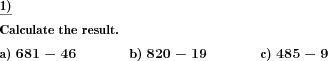Custom math worksheets at your fingertips# Details for problem "Subtraction two numbers with specific no of places"

Quickname: 9801

Elementary School, Primary School, Junior High School, Middle School.

## Summary

Subtraction of two numbers with controlled number of digits for each number.

## Example## Description

One of the basic and most important topics of Maths is a subtraction that is a base for further studies as well as in the real world. Two positive natural numbers with no decimal places have to be subtracted one from another. Use this problem after learners are comfortable with column subtraction with/ without borrowing to practice subtraction deeply. This type of problem is best and customizable for independent practice to learn and master all types of subtraction starting from single digit and up to six-digit no of places.

Develop the following skills by practicing these worksheets.

1. Know the subtraction in equation form.
2. Use of borrowing numbers.
3. Learn to write given numbers in column form and then subtract two numbers.
4. Make grounds for use of subtraction in future Maths.
5. Learn the subtraction skills to apply in real-world problems.

These worksheets can be generated in a variety of ways to achieve learner’s mastery in subtraction for grades 1 to 6.

• The number of digits for the first number (subtrahend) and the second number (minuend) can be chosen from 1 to 6.
• It may be specified whether a borrow over the leftmost place of the second number is allowed.
• The number of problems can be selected.

Download free printable worksheets for this math problem here. The worksheet contains the problems only, the solution sheet includes the answers. Just click on the respective link.

•Worksheet 1Solution sheet with answers
•Worksheet 2Solution sheet with answers
•Worksheet 3Solution sheet with answers

If you can not see the solution sheets for download, they may be filtered out by an ad blocker that you may have installed. If this is the case, please allow ads for this page and reload the page. The solution sheets will then reappear.

• Do these sample worksheets do not really fit?
• Do you need more math worksheets, with a different level of difficulty?
• Would you like to combine different problems on a worksheet and adjust them to your needs?
• As a teacher, you can put together your own worksheets using the automatically generated math problems provided.
With a free initial credit, you can start creating your own math worksheets in a few minutes.

You can try it for free! Register here, to create custom worksheets now!

## Customization options for this problem

Parameter
Possible values
Number of problems
1, 2, 3, 4, 5, 6, 7, 8, 9, 10
Minuend places
1, 2, 3, 4, 5, 6
Subtrahend places
1, 2, 3, 4, 5, 6
Borrows
Never, May, Always

## Similar problems

Remark
Description
Variant in the form of a matrix
Table of addition and subtraction problems